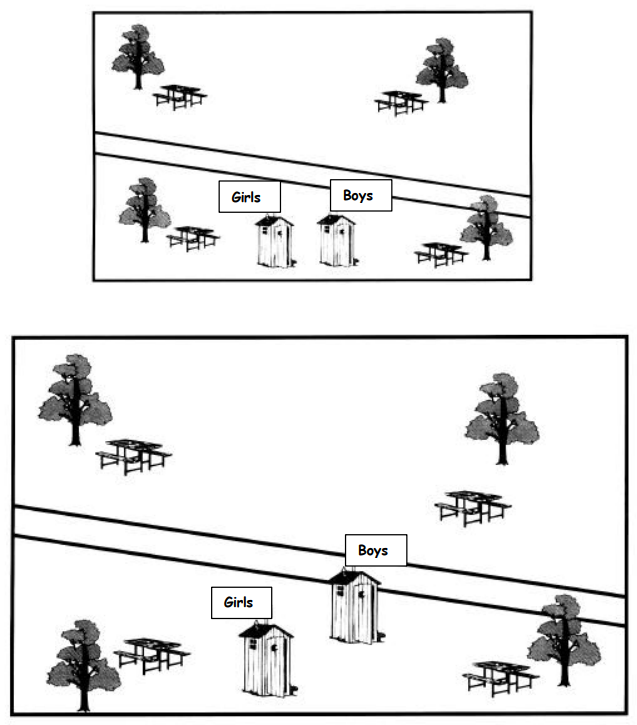Subject:
Mathematics

Topics: Ratios
Common Core State Standard: 6.RP.3, 7.RP.1,
Concepts:

· Ratio

Knowledge and Skills:

· Can measure lengths
· Can use proportional reasoning to solve problems

Lesson:

Procedure: Students can do this activity individually or in teams of two. Each student or team will need a centimeter ruler.

Hand out the activity sheet and discuss the two maps. Explain that one map is supposed to be an exact copy of the other map, only larger in the ratio of 3:2. As a class activity, have all students measure the lengths and widths of the two maps and verify that they are in the correct ratio.

Explain that there are three items in the bottom map which are not in the correct position. Tell students that their assignment is to find out which items those are, and to figure out where they should be. Ensure that they understand the problem, but do not allow discussion of solutions yet. Once all students do understand the problem, have them start trying to solve it.

Circulate as students work on the problem. If needed, you may give individual students or teams the hint that they could measure the distance of all objects from two of the corners of the maps. (The distances should be measured in millimeters as this makes it easiest to compare them.)

Once students discover the three items that are out of place (the tree at bottom left, the boys outhouse, and the picnic table at upper right), they will need to carefully measure the positions of those items on the smaller map (by measuring the distance to any two corners), and then determine the corresponding distances on the larger map using the ratio of 3:2.

In addition to improving students grasp of the concept of ratio and their ability to work with ratios, students will gain some experience with the idea that the position of any object on a two dimensional surface can be specified by making two measurements.

### The Campground

The campground map at the bottom is supposed to be a copy of the map at the top, only larger in the same ratio as 3:2. But there are three items in the bottom map that are not in the right position. Can you tell what they
are and show where they should be?